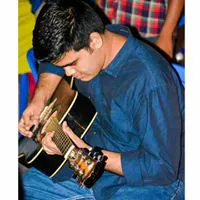# Arduino Based Security System

Do you want to build an easy security system? Well this is the perfect tutorial for you!

BeginnerFull instructions provided1 hour10,210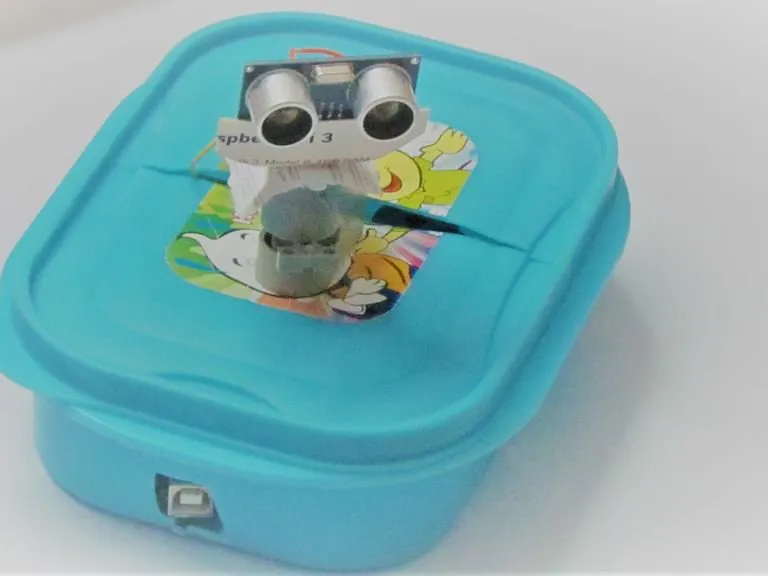## Things used in this project

### Hardware componentsArduino UNO & Genuino UNO
×1Ultrasonic Sensor - HC-SR04 (Generic)
×1
 Servos (Tower Pro MG996R)
×1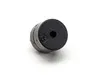Buzzer
×1×1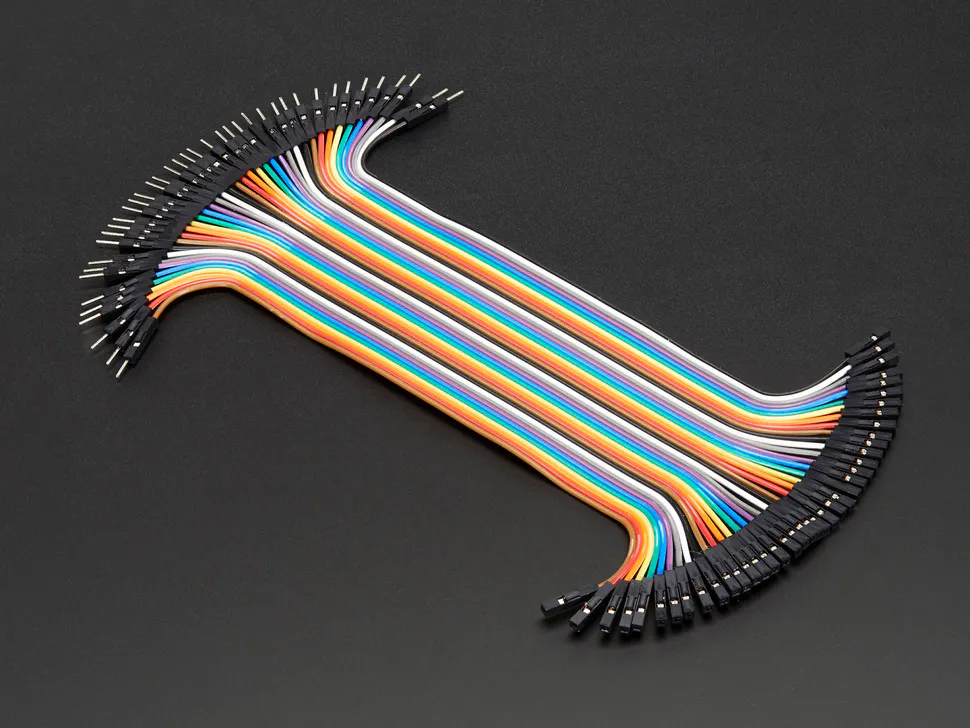Male/Female Jumper Wires
×1
 Plastic Box
×1

### Software apps and online servicesArduino IDE
 Processing IDE

 Knife

## Schematics

### ultrasonic_with_buzzer_bb_K21QkUrwkn.png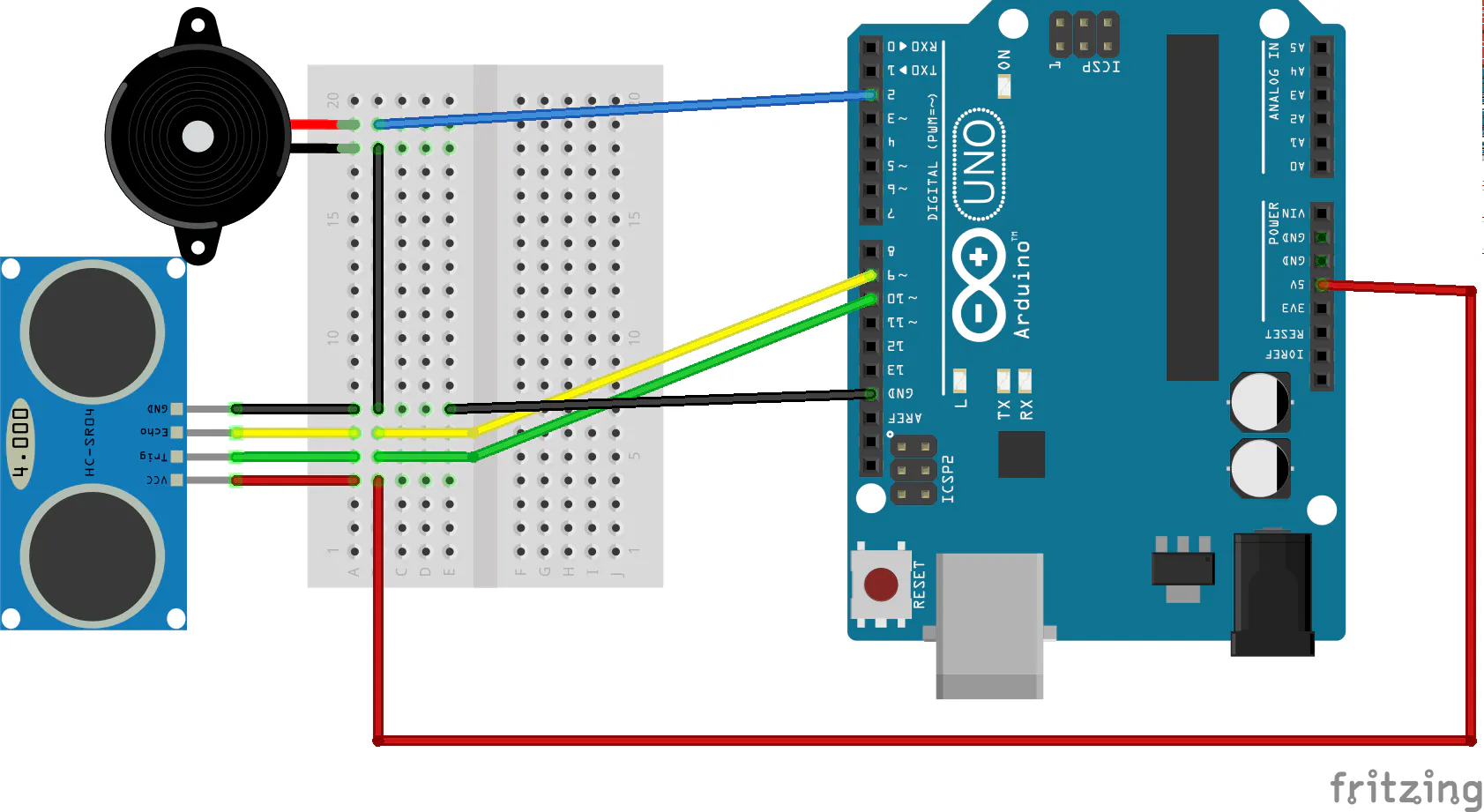## Code

### Security System_IDE

C9Search
```#include <Servo.h>.
int tPin = 2;
int ePin = 3;
int buz = 10;
int d1,d2;
int i =0;
int sdistance;
Servo myServo;
void setup() {
Serial.begin(9600);
pinMode(tPin, OUTPUT);
pinMode(ePin, INPUT);
pinMode(buz,OUTPUT);
myServo.attach(9);
}
void loop() {
for(i=0;i<=180;i += 10)
{
myServo.write(i);
delay(30);
d2 = calculateDistance();

Serial.print(i);
Serial.print(",");
Serial.print(d2);
Serial.print(".");
}

for(i=180;i>0; i-=10){
myServo.write(i);
delay(30);
d2 = calculateDistance();
Serial.print(i);
Serial.print(",");
Serial.print(d2);
Serial.print(".");
}
}
int calculateDistance(){

digitalWrite(tPin,HIGH);
delay(1000);
digitalWrite(tPin, LOW);
d1 = pulseIn(ePin, HIGH);
d2 = d1 * 0.0171821306;
if (d2 < 10)
{
digitalWrite(buz,HIGH);

}
else
{
digitalWrite(buz,LOW);
}
return d2;

}
```

### Processing

Java
```import processing.serial.*; // imports library for serial communication
import java.awt.event.KeyEvent; // imports library for reading the data from the serial port
import java.io.IOException;
Serial myPort; // defines Object Serial
// defubes variables
String angle="";
String distance="";
String data="";
String noObject;
float pixsDistance;
int iAngle, iDistance;
int index1=0;
int index2=0;
PFont orcFont;
void setup() {

size (1366, 768); // ***CHANGE THIS TO YOUR SCREEN RESOLUTION***
smooth();
myPort = new Serial(this,"COM3", 9600); // starts the serial communication
myPort.bufferUntil('.'); // reads the data from the serial port up to the character '.'. So actually it reads this: angle,distance.
}
void draw() {

fill(98,245,31);
// simulating motion blur and slow fade of the moving line
noStroke();
fill(0,4);
rect(0, 0, width, height-height*0.065);

fill(98,245,31); // green color
// calls the functions for drawing the radar
drawLine();
drawObject();
drawText();
}
void serialEvent (Serial myPort) { // starts reading data from the Serial Port
// reads the data from the Serial Port up to the character '.' and puts it into the String variable "data".
data = data.substring(0,data.length()-1);

index1 = data.indexOf(","); // find the character ',' and puts it into the variable "index1"
angle= data.substring(0, index1); // read the data from position "0" to position of the variable index1 or thats the value of the angle the Arduino Board sent into the Serial Port
distance= data.substring(index1+1, data.length()); // read the data from position "index1" to the end of the data pr thats the value of the distance

// converts the String variables into Integer
iAngle = int(angle);
iDistance = int(distance);
}
pushMatrix();
translate(width/2,height-height*0.074); // moves the starting coordinats to new location
noFill();
strokeWeight(2);
stroke(98,245,31);
// draws the arc lines
arc(0,0,(width-width*0.0625),(width-width*0.0625),PI,TWO_PI);
arc(0,0,(width-width*0.27),(width-width*0.27),PI,TWO_PI);
arc(0,0,(width-width*0.479),(width-width*0.479),PI,TWO_PI);
arc(0,0,(width-width*0.687),(width-width*0.687),PI,TWO_PI);
// draws the angle lines
line(-width/2,0,width/2,0);
popMatrix();
}
void drawObject() {
pushMatrix();
translate(width/2,height-height*0.074); // moves the starting coordinats to new location
strokeWeight(9);
stroke(255,10,10); // red color
pixsDistance = iDistance*((height-height*0.1666)*0.025); // covers the distance from the sensor from cm to pixels
// limiting the range to 40 cms
if(iDistance<10){
// draws the object according to the angle and the distance
}
popMatrix();
}
void drawLine() {
pushMatrix();
strokeWeight(9);
stroke(30,250,60);
translate(width/2,height-height*0.074); // moves the starting coordinats to new location
popMatrix();
}
void drawText() { // draws the texts on the screen

pushMatrix();
if(iDistance>10) {
noObject = "Out of Range";
}
else {
noObject = "In Range";
}
fill(0,0,0);
noStroke();
rect(0, height-height*0.0648, width, height);
fill(98,245,31);
textSize(25);

text("2cm",width-width*0.3854,height-height*0.0833);
text("4cm",width-width*0.281,height-height*0.0833);
text("6cm",width-width*0.177,height-height*0.0833);
text("10cm",width-width*0.0729,height-height*0.0833);
textSize(40);
text("Akshay6766 ", width-width*0.875, height-height*0.0277);
text("Angle: " + iAngle +" ", width-width*0.48, height-height*0.0277);
text("Distance: ", width-width*0.26, height-height*0.0277);
if(iDistance<40) {
text("        " + iDistance +" cm", width-width*0.225, height-height*0.0277);
}
textSize(25);
fill(98,245,60);
text("30",0,0);
resetMatrix();
text("60",0,0);
resetMatrix();
text("90",0,0);
resetMatrix();
text("120",0,0);
resetMatrix();